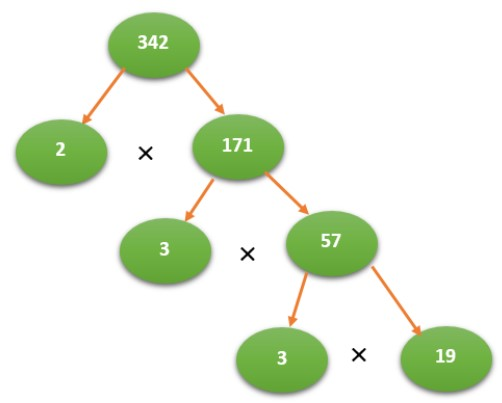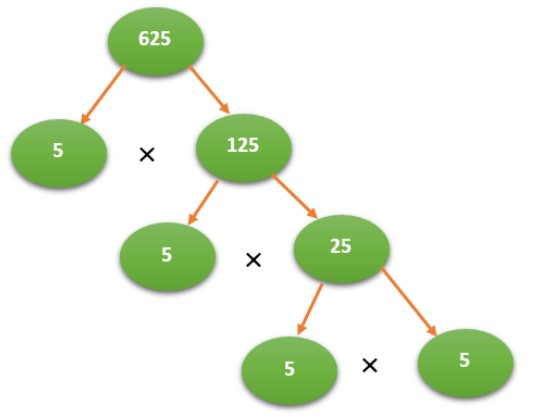# Prime Factorization Calculator

Insert the value and click calculate button to get the prime factors by using this prime factorization calculator.

Give Us Feedback

## Prime Factorization Calculator

Prime factorization calculator is a tool that is used to calculate the prime factors of a given number.

## What is meant by prime factorization?

Prime factorization is a mathematical process that involves decomposing a positive integer into its prime factors. Prime factors are the prime numbers that, when multiplied together, result in the original number. To determine the prime factorization of a number, you identify the prime numbers that divide the given number evenly.

## How to find prime factors?

Example 1:

Determine the prime factors of 342.

Solution

The Prime Factorization is:

2 × 3 × 3 × 19

In Exponential Form:

342 = 2 × 32 × 19

CSV Format:

2, 3, 3, 19

The Prime Factor Tree is:Example 2:

Determine the prime factors of 625.

Solution

Step 1:

The Prime Factorization is:

5 × 5 × 5 × 5

Step 2:

In Exponential Form:

625 = 54

CSV Format:

5, 5, 5, 5

Step 3:

The Prime Factor Tree is:### Other Languages